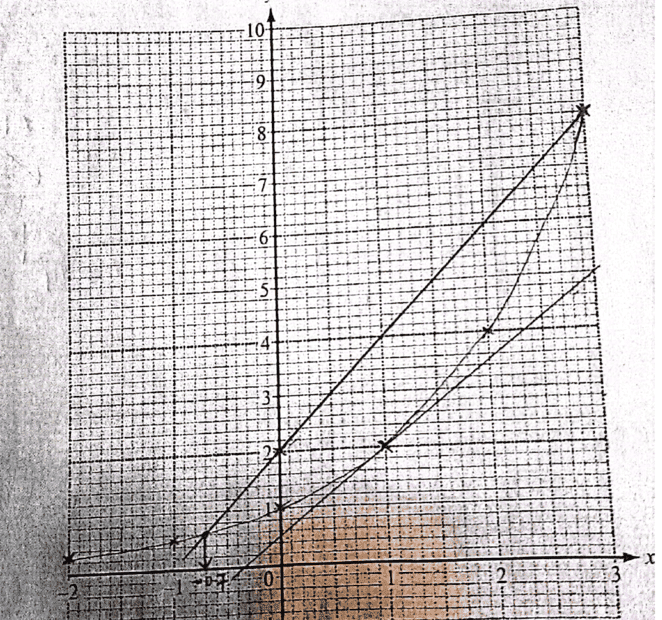# Use the tangent line to estimate the gradient of ##y=2^x## when ##x=1##

Gold Member
Homework Statement:
Draw the tangent to the curve at the point where ##x=1##. Use this tangent to claculate an estimate to the gradient of ##y=2^x## when ##x=1##
Relevant Equations:
Ok this is a question that i am currently marking...the sketch is here;In my mark scheme i have points ##(1,2)## and ##(3,5)## which can be easily picked from the graph to realize an estimate of ##m=1.5## where ##m## is the gradient ...of course i have given a range i.e ##1.6≥m≥1.2##

Now to my question. hmmmmmA student picked the points ##(1,2)## and ##(0.9,1.8)## getting ##m=2## ...the difference from actual is quite big...but the points are picked from their straight line...am i missing something here...

Actual gradient using differentiation would be given by;

##\dfrac{dy}{dx}= 2^x\ln 2##

##\dfrac{dy}{dx}[x=1]= 2^1\ln 2=1.386##

Last edited:

Homework Helper
2022 Award
Is the tangent line drawn by the student, or is it printed on the paper?

In any case, I think $(0.9, 1.8)$ is visibly below the tangent line in the picture.

Gold Member
Is the tangent line drawn by the student, or is it printed on the paper?

In any case, I think $(0.9, 1.8)$ is visibly below the tangent line in the picture.
Drawn by the student...we would not expect the students to draw this accurately 100%. How do i deal with this? should i increase range of expected values? my thinking is; i cannot penalise the student for having picking those points from their tangent line...and the calculation as per their picked points is correct.

Homework Helper
2022 Award
I dont think (0.9, 1.8) is on their tangent line: it's visibly below it. That should result in some loss of marks. If they'd gone with (0.9, 1.85) then they might have a better case.

Gold Member
I just checked the accurate graph on desmos...points ##(0.9,1.85)## would have been fine...

Gold Member
I dont think (0.9, 1.8) is on their tangent line: it's visibly below it. That should result in some loss of marks. If they'd gone with (0.9, 1.85) then they might have a better case.
Thanks...i agree...i will emphasis the need to try and pick obvious points on the graphs to mitigate this. Cheers great weekend mate!

Homework Helper
An additional comment: as a physicist, I would emphasize the large error introduced by picking such a small ##\Delta x##: any error in ##\Delta y## as read off from the graph is multiplied by 10 !

##\ ##

•chwala
Gold Member
Notice in general, unless your function is itself linear to start with, the approximation is only local. But if youre just given the point (1,2), there are infinitely-many lines that go through it. The point (0.9, 1.8) defines just one of infinitely-many such lines. Not sure if this addresses your question.

Gold Member
Notice in general, unless your function is itself linear to start with, the approximation is only local. But if youre just given the point (1,2), there are infinitely-many lines that go through it. The point (0.9, 1.8) defines just one of infinitely-many such lines. Not sure if this addresses your question.
Not sure ...we need points that are on the tangent line and as discussed above, it's clear that ##(0.9,1.8)## is not a point on the tangent line to the given curve ##y=2^x##.

Gold Member
Not sure ...we need points that are on the tangent line and as discussed above, it's clear that ##(0.9,1.8)## is not a point on the tangent line to the given curve ##y=2^x##.
Do you mean at the point (1,2)?

Gold Member
Do you mean at the point (1,2)?
Yes.